# Programming with ggplot2

## Introduction

A major requirement of a good data analysis is flexibility. If your data changes, or you discover something that makes you rethink your basic assumptions, you need to be able to easily change many plots at once. The main inhibitor of flexibility is code duplication. If you have the same plotting statement repeated over and over again, you’ll have to make the same change in many different places. Often just the thought of making all those changes is exhausting!

To make your code more flexible, you need to reduce duplicated code by writing functions. When you notice you’re doing the same thing over and over again, think about how you might generalise it and turn it into a function. If you’re not that familiar with how functions work in R, you might want to brush up your knowledge at http://adv-r.had.co.nz/Functions.html.

In this chapter I’ll show how to write functions that create:

• A single ggplot2 component.
• Multiple ggplot2 components.
• A complete plot

And then I’ll finisih off with a brief illustration of how you can apply functional programming techniques to ggplot2 objects.

You might also find the cowplot and ggthemes packages helpful. As well as providing reuable components that help you directly, you can also read the source code of the packages to figure out how they work.

## Single components

Each component of a ggplot plot is an object. Most of the time you create the component and immediately add it to a plot, but you don’t have to. Instead, you can save any component to a variable (giving it a name), and then add it to multiple plots:

``````bestfit <- geom_smooth(
method = "lm",
se = FALSE,
colour = alpha("steelblue", 0.5),
size = 2
)
ggplot(mpg, aes(cty, hwy)) +
geom_point() +
bestfit
ggplot(mpg, aes(displ, hwy)) +
geom_point() +
bestfit``````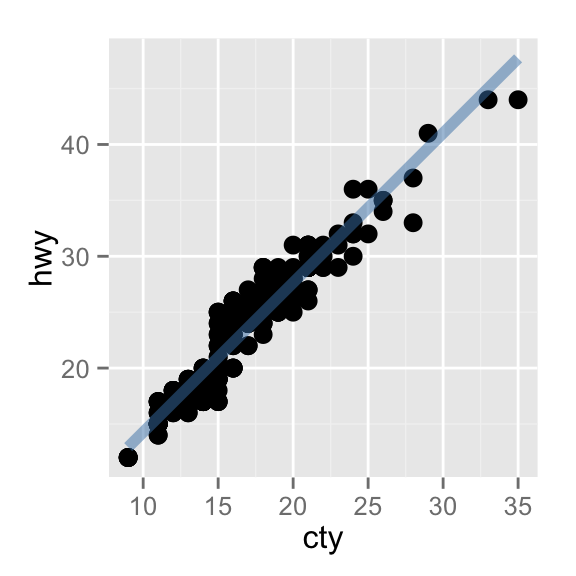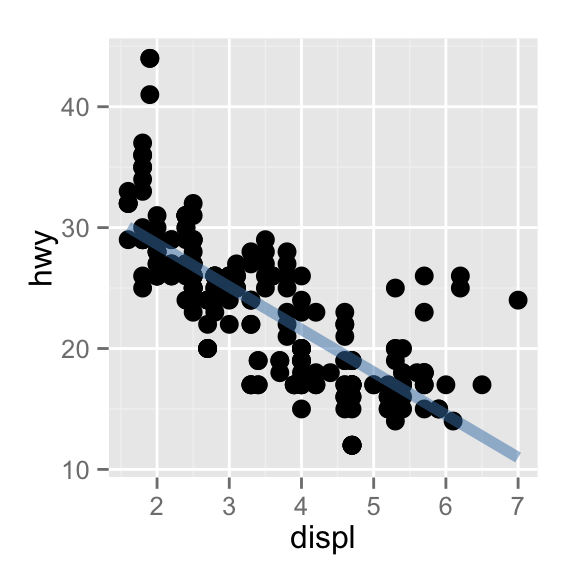That’s a great way to reduce simple types of duplication (it’s much better than copying-and-pasting!), but requires that the component be exactly the same each time. If you need more flexibility, you can wrap these reusable snippets in a function. For example, we could extend our `bestfit` object to a more general function for adding lines of best fit to a plot. The following code creates a `geom_lm()` with three parameters: the model `formula`, the line `colour` and the line `size`:

``````geom_lm <- function(formula = y ~ x, colour = alpha("steelblue", 0.5),
size = 2, ...)  {
geom_smooth(formula = formula, se = FALSE, method = "lm", colour = colour,
size = size, ...)
}
ggplot(mpg, aes(displ, 1 / hwy)) +
geom_point() +
geom_lm()
ggplot(mpg, aes(displ, 1 / hwy)) +
geom_point() +
geom_lm(y ~ poly(x, 2), size = 1, colour = "red")``````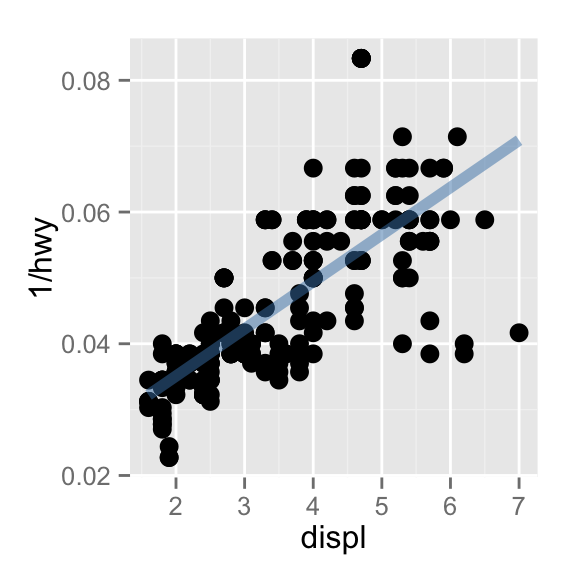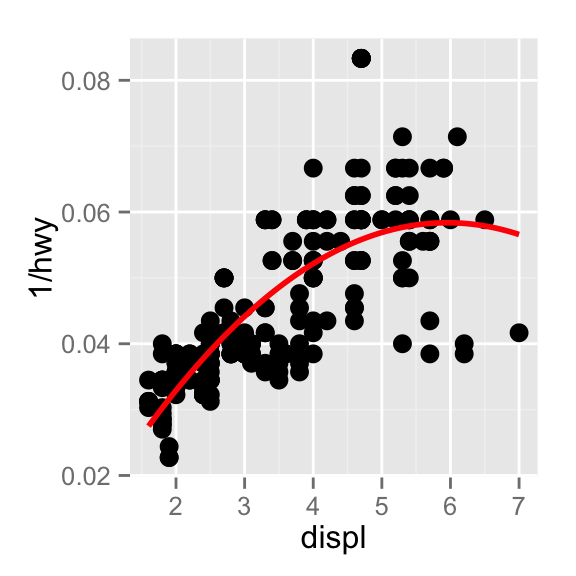Note the use of `...`. When included in the function definition `...` allows a function to accept arbitrary additional arguments. Inside the function, you can then use `...` to pass those arguments on to another function. Here we pass `...` onto `geom_smooth()` so the user can still modify all the other arguments we haven’t explicit overridden. When you write your own component functions, it’s a good idea to always use `...` is this way.

### Exercises

1. Create an object that represents a pink histogram with 100 bins.

2. Create an object that represents a fill scale with the Blues ColorBrewer palette.

3. Read the source code for `theme_grey()`. What are its arguments? How does it work?

4. Create `scale_colour_wesanderson()`. It should have a parameter to pick the palette from the wesanderson package, and create either a continuous or discrete scale.

## Multiple components

It’s not alway possible to achieve your goals with a single componens. Fortunately, ggplot2 has a convenient way of adding multiple components to a plot in one step with a list. The following function adds two layers: one to show the mean, and one to show its standard error:

``````geom_mean <- function() {
list(
stat_summary(fun.y = "mean", geom = "bar", fill = "grey70"),
stat_summary(fun.data = "mean_cl_normal", geom = "errorbar", width = 0.4)
)
}
ggplot(mpg, aes(class, cty)) + geom_mean()
#> Warning: replacing previous import by 'scales::alpha' when loading 'Hmisc'
ggplot(mpg, aes(drv, cty)) + geom_mean()``````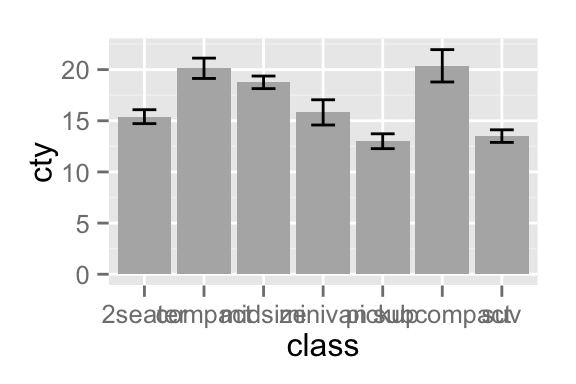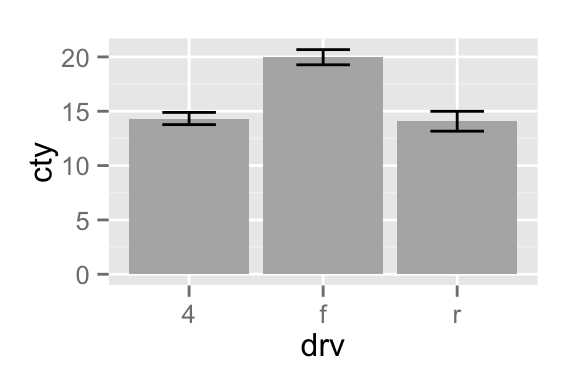If the list contains any `NULL` elements, they’re ignored. This makes it easy to conditionally add components:

``````geom_mean <- function(se = TRUE) {
list(
stat_summary(fun.y = "mean", geom = "bar", fill = "grey70"),
if (se)
stat_summary(fun.data = "mean_cl_normal", geom = "errorbar", width = 0.4)
)
}
ggplot(mpg, aes(class, cty)) + geom_mean()
ggplot(mpg, aes(class, cty)) + geom_mean(se = FALSE)``````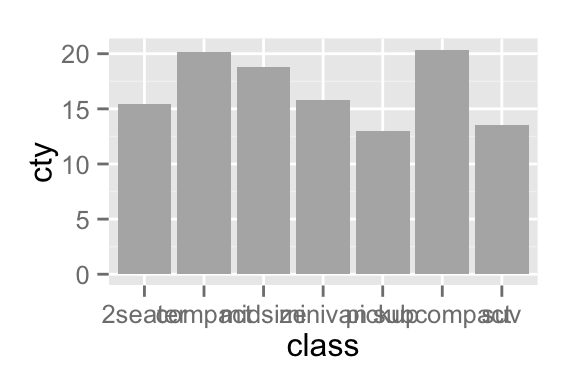### Plot components

You’re not just limited to adding layers in this way. You can also include any of the following object types in the list:

• A data.frame, which will override the default dataset associated with the plot. (If you add a data frame by itself, you’ll need to use `%+%`, but this is not necessary if the data frame is in a list)

• An `aes()` object, which will combined with the existing default aesthetic mapping.

• Scales, which override existing scales, with a warning if they’ve already been set by the user.

• Coordinate systems and facetting specification, which override the existing settings.

• Theme components, which override the specified components.

### Annotation

It’s often useful to add standard annotations to a plot. In this case, your function will also set the data in the layer function, rather than inheriting it from the plot. There are two other options that you should set when you do this. These ensure that the layer is self-contained:

• `inherit.aes = FALSE` presents the layer from inheriting aesthetics from the parent plot. This ensures your annotation works regardless of what else is on the plot.

• `show.legend = FALSE` ensuresthat your annotation won’t appear in the legend.

One example of this technique is the `borders()` function built into ggplot2. It’s designed to add map borders from one of the dataset in the maps package:

``````borders <- function(database = "world", regions = ".", fill = NA,
colour = "grey50", ...) {
df <- map_data(database, regions)
geom_polygon(
aes_(~lat, ~long, group = ~group),
data = df, fill = fill, colour = colour, ...,
inherit.aes = FALSE, show.legend = FALSE
)
}``````

### Additional arguments

If you want to pass additional arguments to the components in your function, `...` is no: there’s no way to direct different arguments to different components. Instead, you’ll need to think about how you want your function to work, balancing the benefits of having one function that does it all vs. the cost of having a complex function that’s harder to understand.

To get your start, here’s one approach using `modifyList()` and `do.call()`:

``````geom_mean <- function(..., bar.params = list(), errorbar.params = list()) {
params <- list(...)
bar.params <- modifyList(params, bar.params)
errorbar.params  <- modifyList(params, errorbar.params)

bar <- do.call("stat_summary", modifyList(
list(fun.y = "mean", geom = "bar", fill = "grey70"),
bar.params)
)
errorbar <- do.call("stat_summary", modifyList(
list(fun.data = "mean_cl_normal", geom = "errorbar", width = 0.4),
errorbar.params)
)

list(bar, errorbar)
}

ggplot(mpg, aes(class, cty)) +
geom_mean(
colour = "steelblue",
errorbar.params = list(width = 0.5, size = 1)
)
ggplot(mpg, aes(class, cty)) +
geom_mean(
bar.params = list(fill = "steelblue"),
errorbar.params = list(colour = "blue")
)``````